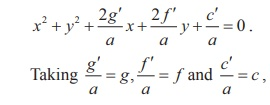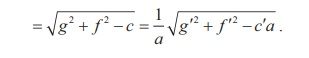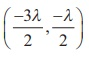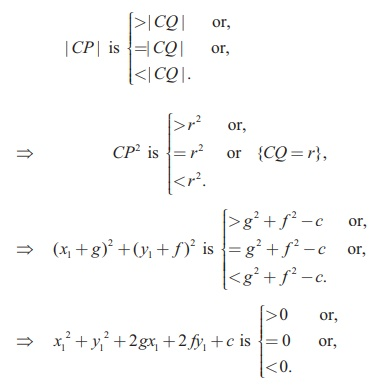Home | | Maths 12th Std | Equation of a circle in standard form

# Equation of a circle in standard form

(i) Equation of circle with centre (0, 0) and radius r (ii) Equation of circle with centre (h, k) and radius r

Equation of a circle in standard form

## (i) Equation of circle with centre (0, 0) and radius r

Let the centre be C(0, 0) and radius be r and P(x, y) be the moving point.

Note that the point P having coordinates (x, y) is represented as P(x, y) .

Then, CP  =  r and so CP2 = r2

Therfore (x − 0)2 + ( y − 0)2 = r2That is x2 + y2 = r2

This is the equation of the circle with centre (0, 0) and radius r

## (ii) Equation of circle with centre (h, k) and radius r

Let the centre be C(h, k ) and r be the radius and P(x, y) be the moving point,

Then, CP = r and so CP2 = r2 .

That is, (x h)2 + ( y k )2 = r2 .This is the equation of the circle in Standard form, which is also known as centre-radius form.

Expanding the equation, we get

x2 + y2 − 2hx − 2ky + h2 + k2r2 = 0 .

Taking 2g = −2h, 2 f = −2k, c = h2 + k2r2 , the equation takes the form

x2 + y2 + 2gx + 2 fy + c = 0 , called the general form of a circle.The equation x2 + y2 + 2gx + 2 fy + c = 0 is a second degree equation in x and y possessing the following characteristics:

(i) It is a second degree equation in x and y ,

(ii) coefficient of x2 = coefficient of y2 ≠ 0 ,

(iii) coefficient of xy = 0 .

Conversely, we prove that an equation possessing these three characteristics, always represents a circle. Let

ax2  + ay2  + 2gx + 2 f y′  + c = 0 ………………………………(1)

be a second degree equation in x and y having characteristics (i), (ii) and (iii), a ≠ 0 . Dividing (1) by a, givesequation (2) becomes x2 + y2 + 2gx + 2 fy + c = 0 .

Adding and subtracting g2 and f2 , we get x2 + 2gx + g2 + y2 + 2 fy + f 2 - g2 - f 2 + c = 0

(x + g)2 + ( y + f )2 = g 2 + f 2c

(x - (-g))2  + ( y - (- f ))2  = ( √[ g2  +  f 2  - c] )2

This is in the standard form of a circle with centre C (-g, - f ) and radius r = √[ g2  +  f 2  - c]. Hence equation (1) represents a circle with centre (-g, - f ) = ( -g’/a , - f’/a ) and radiusNote

The equation x2 + y2 + 2gx + 2 fy + c = 0 represents a circle with centre (–g, f ) and radiu √[ g2  +  f 2  - c].

(i) It is a real circle if g2 + f2 - c > 0 ;

(ii) a point circle if g2 + f 2 - c = 0 ;

(iii) or an imaginary circle if g2 + f 2 - c < 0 with no locus.

Example 5.1

Find the general equation of a circle with centre (−3, −4) and radius 3 units.

Solution

Equation of the circle in standard form is  ( x - h)2  + ( y - k )2  = r 2

(x - (-3))2  + ( y - (-4))2  = 32

( x + 3)2  + ( y + 4)2  = 32

x2 + y2 + 6x + 8 y +16 = 0 .

Theorem 5.1

The circle passing through the points of intersection (real or imaginary) of the line lx + my + n = 0 and the circle x2 + y2 + 2gx + 2 fy + c = 0 is the circle of the form

x2 + y2 + 2gx + 2 fy + c + λ (lx + my + n) = 0 , λ R1.

Proof

Let the circle be S : x2 + y2 + 2gx + 2 fy = 0 , … (1)

and the line be L : lx + my + n = 0 . … (2)

Consider  S + λL = 0  .  That is  x2  + y2  + 2gx + 2fy + c +λ(lx + my + n) = 0    ... (3)

Grouping the terms of x, y and constants, we get

x2  + y2  + x(2g +λl)+ y(2fm)+ c +λn = 0 which is a second degree equation in x and y with coefficients of x2 and y2 equal and there is no xy term.

If (α, β) is a point of intersection of S and L satisfying equation (1) and (2), then it satisfies equation (3).

Hence  S + λL = 0  represents the required circle.

Example 5.2

Find the equation of the circle described on the chord 3x + y + 5 = 0 of the circle x2 + y2 = 16 as diameter.

Solution

Equation of the circle passing through the points of intersection of the chord and circle by Theorem 5.1 is x2 + y2 -16 + l (3x + y + 5) = 0 .

The chord 3x + y + 5 = 0 is a diameter of this circle if the centrelies on the chord.Therefore, the equation of the required circle is x2 + y2 + 3x + y -11 = 0.

Example 5.3

Determine whether x + y −1 = 0 is the equation of a diameter of the circle x2 + y2 − 6x + 4 y + c = 0 for all possible values of c .

Solution

Centre of the circle is (3, -2) which lies on x + y -1 = 0 . So the line x + y -1 = 0 passes through the centre and therefore the line x + y -1 = 0 is a diameter of the circle for all possible values of c .

Theorem 5.2

The equation of a circle with (x1 , y1 ) and (x2 , y2 ) as extremities of one of the diameters of the circle is (x x1 )(x x2 ) + ( y y1)( y y2) = 0.

Proof

Let A(x1 , y1 ) and B(x2 , y2 ) be the two extremities of the diameter AB , and P(x, y) be any point on the circle. Then ÐAPB = π/2 (angle in a semi-circle).Therefore, the product of slopes of AP and PB is equal to -1.

That is,= −1 yielding the equation of the required circle as

(x - x1 )(x - x2 ) + ( y - y1 )( y - y2 ) = 0 .

Example 5.4

Find the general equation of the circle whose diameter is the line segment joining the points (−4, −2) and (1,1) .

Solution

Equation of the circle with end points of the diameter as( x1 , y1 ) and ( x2 , y2 ) given in

theorem 5.2 is

( x - x1 )( x - x2 ) + ( y - y1 )( y - y2 ) = 0

( x + 4)( x -1) + ( y + 2)( y -1) = 0

x2 + y2 + 3x + y - 6 = 0 which is the required equation of the circle.

Theorem 5.3

The position of a point P(x1 , y1 ) with respect to a given circle x2 + y2 + 2gx + 2 fy + c = 0 in the Plane containing the circle is Outside or on or inside the circle according as x2 + y2 + 2gx + 2 fy + c isProof

Equation of the circle is x2 + y2 + 2gx + 2 fy + c = 0 with centre C (-g, - f ) and radius rLet P(x1, y1 ) be a point in the plane. Join CP and let it meet the circle at Q .Then the point P is outside, on or within the circle according asExample 5.5

Examine the position of the point (2, 3) with respect to the circle x2 + y2 − 6x − 8 y +12 = 0.

Solution

Taking (x1, y1) as (2, 3), we get

x2 + y2 + 2gx1 + 2 fy1 + c = 22 + 32 - 6 ´ 2 - 8´ 3 +12 ,

= 4 + 9 -12 - 24 + 12 = -11 < 0.

Therefore, the point (2, 3) lies inside the circle, by theorem 5.3.

Example 5.6

The line 3x + 4 y −12 = 0 meets the coordinate axes at A and B . Find the equation of the circle drawn on AB as diameter.

Solution

Writing the line 3x + 4y = 12, in intercept form yields x/4 + y/3 = 1. Hence the points A and B are (4, 0) and(0, 3) .

Equation of the circle in diameter form is

( x - x1 )( x - x2 ) + ( y - y1 )( y - y2 ) = 0

( x - 4)( x - 0) + ( y - 0)( y - 3) = 0

x2 + y2 - 4x - 3y = 0

Example 5.7

A line 3x + 4 y +10 = 0 cuts a chord of length 6 units on a circle with centre of the circle (2,1) . Find the equation of the circle in general form.

Solution

C(2,1) is the centre and 3x + 4 y +10 = 0 cuts a chord AB on the circle. Let M be the midpoint of AB . Then we have

AM  = BM = 3 . Now  BMC is a right triangle.

So, we have CM  == 4.

By Pythogoras theorem BC2 = BM2 + MC2  = 32 + 42 = 25So, the equation of the required circle is (x - 2)2 + ( y -1) = 52

x2 + y2 - 4x - 2 y – 20 = 0.

Example 5.8

A circle of radius 3 units touches both the axes. Find the equations of all possible circles formed in the general form.

Solution

As the circle touches both the axes, the distance of the centre from  both the axes is 3 units, centre can be (±3, ±3) and hence there are four circles with radius 3, and the required equations of the four circles are x2 + y2 ± 6x ± 6 y + 9 = 0 .Example 5.9

Find the centre and radius of the circle 3x2 + (a +1) y2 + 6x - 9y + a + 4 = 0.

Solution

Coefficient of x2 = Coefficient of y2 (characteristic (ii) for a second degree equation to represent a circle).

That is, 3 = a +1 and a = 2 .

Therefore, the equation of the circle is

3x2 + 3y2 + 6x - 9 y + 6 = 0

x2 + y2 + 2x - 3y + 2 = 0

So, centre is ( -1, 3/2 ) and radius r =Example 5.10

Find the equation of the circle passing through the points (1,1), (2, −1) , and (3, 2) .

Solution

Let the general equation of the circle be

x2 + y2 + 2gx + 2 fy + c = 0        ……….(1)

It passes through points (1,1), (2, -1) and (3, 2) .

Therefore, 2g + 2 f + c = -2 , … (2)Therefore, the required equation of the circle isÞ x2 + y2 - 5x - y + 4 = 0

Note

Three points on a circle determine the equation to the circle uniquely. Conversely three equidistant points from a centre point forms a circle.

Tags : Formula, Solved Example Problems , 12th Mathematics : UNIT 5 : Two Dimensional Analytical Geometry II
Study Material, Lecturing Notes, Assignment, Reference, Wiki description explanation, brief detail
12th Mathematics : UNIT 5 : Two Dimensional Analytical Geometry II : Equation of a circle in standard form | Formula, Solved Example Problems